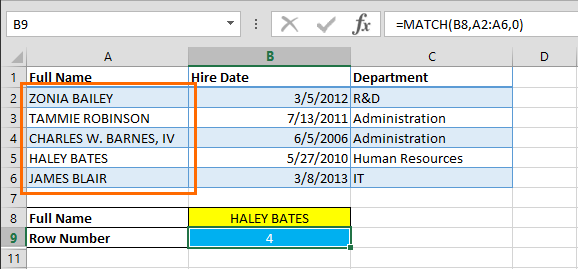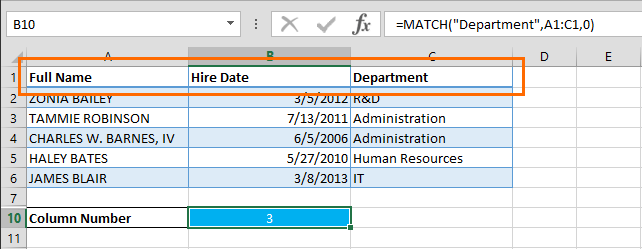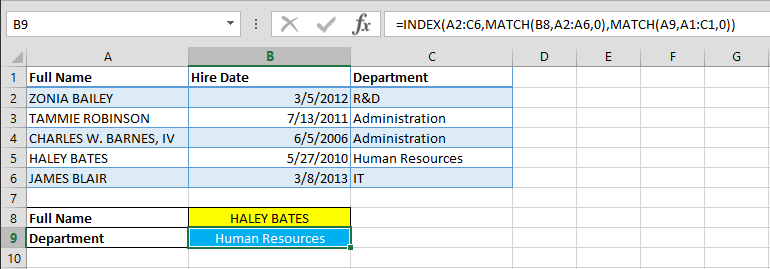VLOOKUP and HLOOKUP are the go-to functions for most Excel users to look up data in a table. However, there is an alternative to this function that can do the same job, with added benefits. It’s the INDEX and MATCH combo. Using these two formulas in conjunction can give you more flexibility when searching for data.

The idea behind INDEX & MATCH is simple, INDEX function finds the data reference, the MATCH function searches for the desired value in an array (single dimensional), and returns the coordinates as a number. The numbers fed by the MATCH function essentially becomes the coordinates for the INDEX function and voila!

## INDEX

The syntax is as follows,

=INDEX(array, row_num, [column_num])

Parameters:

• array: the look up table
• row_num: row coordinate
• column_num: column coordinate (optional)

For example, the INDEX formula below will return the value in the 4th row and 3rd column in the A2:C6 range,

=INDEX(A2:C6,4,3)INDEX function can work with single dimensional ranges without an column_num argument. The same goes for horizontal single dimensional ranges. Here is an example for a vertical single dimensional range,

=INDEX(C2:C6,4)Below is an example for a horizontal range,

=INDEX(A5:C5,3)## MATCH

MATCH function has 3 arguments,

=MATCH(lookup_value, lookup_range, [match_type])

Parameters:

• lookup_value: what you want to look up
• lookup_range: where you are looking
• match_type: match type (optional)

While lookup_value and lookup_range are pretty self-explanatory, match_type can be confusing at first. This parameter essentially works like VLOOKUP and HLOOKUP’s range_lookup and tells Excel to do an exact, or an approximate match. However, instead of range_lookup’s 2-option TRUE/FALSE values, match_type can have three values (-1, 0, and 1).

 Value Match Type Behavior 1 or omitted Approximate MATCH finds the largest value less than or equal to lookup value. Lookup array must be sorted in ascending order. 0 Exact MATCH finds the first value exactly equal to lookup value. Lookup array does not need to be sorted. -1 Approximate MATCH finds the smallest value greater than or equal to lookup value. Lookup array must be sorted in descending order.

Although match_type is optional and your MATCH function will work just fine without it for approximate searches, it is a good practice to always enter a value here. Trying to search an exact value without arguments can give you the wrong results.

Let’s look at an example again. The formula below can give us the position of the employee name entered into B8,

=MATCH(B8,A2:A6,0)To look for the department position,

=MATCH("Department",A1:C1,0)## INDEX & MATCH

Now, let’s combine the two functions. To break it down,

=INDEX(table you are looking,

MATCH(what you want to look up, where you are looking, match type),

MATCH(what you want to look up, where you are looking, match type)

)

If either the row or column coordinate has a static value, you can replace that value with one of the MATCH functions, which in this case is redundant. Combining the two examples from before, let’s look for the employee’s department.

=INDEX(A2:C6,MATCH(B8,A2:A6,0),MATCH(A9,A1:C1,0))

The formula above will highlight these ranges,Searching for ‘Haley Bates’ gave us ‘Human Resources’ as her department.### 2-way lookup

VLOOKUP can only search from left to right, whereas INDEX & MATCH combination allows it to overcome this limitation and look up data both left to right and right to left.

### Performance

Although this may not mean much for casual Excel users, VLOOKUP drastically slow down complicated data models. INDEX & MATCH offer better overall performance.

The combo is a lifesaver when working with array formulas that perform multiple actions with a single formula.

### No issues when inserting or deleting columns (or rows for HLOOKUP)

If column index number (3rd argument of VLOOKUP and HLOOKUP) wasn’t been set dynamically, it’s usually not a good idea to delete or move a column in the lookup table. Plus, setting column index number dynamically means that you’re going to be needing another formula inside VLOOKUP. Yeah, you’re not dealing with a single formula anymore in this case.

The INDEX & MATCH alternative is a safer bet if you think you’re going to be inserting or deleting columns. This becomes a great advantage when working with large datasets that require frequent updates.

### No 255-character limit for a lookup value's size

Both VLOOKUP and MATCH are limited to 255 characters for lookup criteria. However; INDEX & MATCH combo make this search viable with a little tweak. For example, if lookup value (E2) exceeds the 255 character limit, a modified version of INDEX & MATCH combination can be used (Here we are searching in the range A2:B6),

=INDEX(A2:B6,MATCH(TRUE,INDEX(A2:A6=E2,0),0),2)